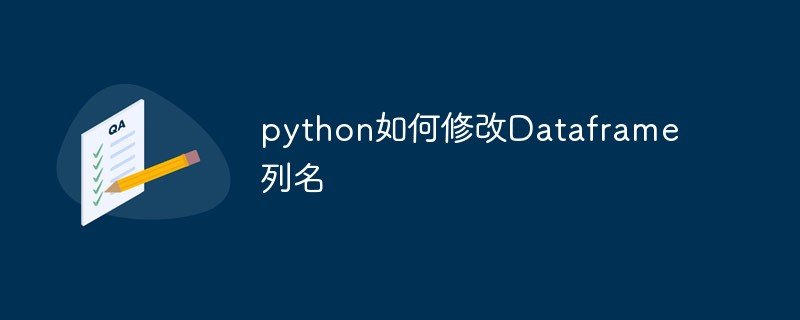# python如何修改Dataframe列名`import pandas as pd`
`df = pd.DataFrame({'a':[1,2,3],'b':[1,2,3]})`

a b
0 1 1
1 2 2
2 3 31、修改列名a，b为A、B。

`df.columns = ['A','B']`

2、只修改列名a为A

`df.rename(columns={'a':'A'})`• 相关标签：python 修改列名
• 相关文章

相关视频